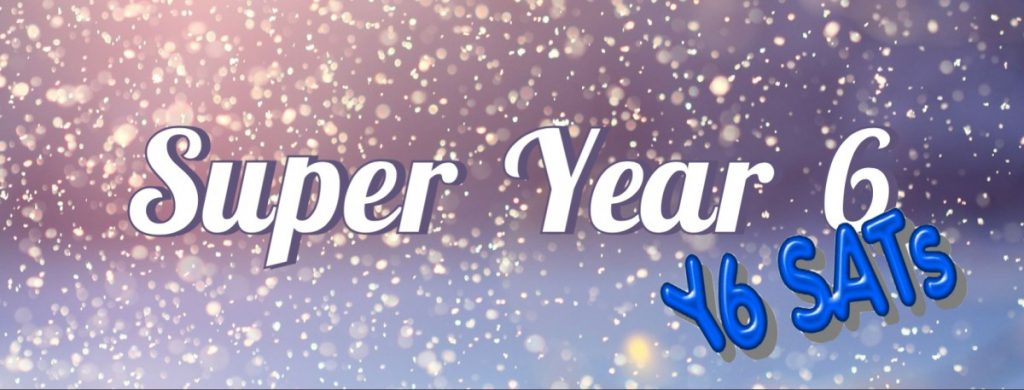### CoordinatesIsabella

1.(2,4) 2. ( 13,15) 3. D=( -10,-4) E=( 0,8) 4. ( 10,9) 5.( 40,27) 6. M=( 15,40) C= ( 20,0) 7. A=( 11,9) B=( 15,3)

Jake

1) (5,2)
2) (13,15)
3) (14,8)
4)
5) (5,1)
6) (11,9)
7) (40,27)
8) (15,40)
9) ( 11,9) (16,3)
10) (3,11)
11) B=(0,10) C=(10,15)
12) (6,28)

Charlie

1.(2,4)
2.(13,15)
3.D.(-10,-4)E.(0,8)
4.Inside,outside,outside,outside.
5.(5,2)
6.(11,9)
7.(38,27)
8.M(15,40)C(20,0)
9.A(11,9)B(15,3)
10.(3,11)
11.A(7,12)B(17,12)
12.B(0,10)C(10,20)
13.(6,28)

Annabelle

My work is on seesaw

Mayon

1. 2,4
2. 10, 15
3. D = -10, 4 E = 0, 8
4. Outside the square
Inside the square
Inside the square
Outside the square
5. D = 5, 2
6. 10, 9
7. 40, 27
8. M = 15, 40 C = 20, 0
9. *
10. *
11. 6, 28

leighton

1.2,4
2,12,12
3.0,-7
4.10,4
11,8
5.6,3Mel Fisher

HLTA

I have had the pleasure of working in primary education for over 20 years, with a keen interest in using I.T., film and animation to enhance learning. I am a mum of two (grown up) boys and love reading crime thrillers.